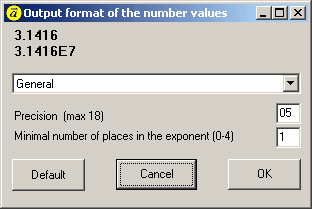Previous  Top  Next

The way of number output can be configurated with a dialog.
Options -> Output f
ormat of numbersThere are 4 different Modes that can be specified with 2 parameter:

General number format The value is converted to the shortest possible decimal string using fixed or scientific format. Trailing zeros are removed from the resulting string, and a decimal point appears only if necessary. The resulting string uses fixed point format if the number of digits to the left of the decimal point in the value is less than or equal to the specified precision, and if the value is greater than or equal to 0.00001. Otherwise the resulting string uses scientific format, and the Digits parameter specifies the minimum number of digits in the exponent (between 0 and 4).

Scientific format. The value is converted to a string of the form "-d.ddd...E+dddd". The resulting string starts with a minus sign if the number is negative, and one digit always precedes the decimal point. The total number of digits in the resulting string (including the one before the decimal point) is given by the Precision parameter. The "E" exponent character in the resulting string is always followed by a plus or minus sign and up to four digits. The 2. parameter specifies the minimum number of digits in the exponent (between 0 and 4).

Fixed point format. The value is converted to a string of the form "-ddd.ddd...". The resulting string starts with a minus sign if the number is negative, and at least one digit always precedes the decimal point. The number of digits after the decimal point is given by the Digits parameter--it must be between 0 and 18. If the number of digits to the left of the decimal point is greater than the specified precision, the resulting value will use scientific format.